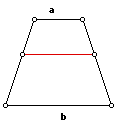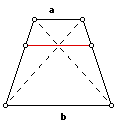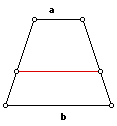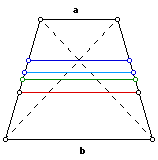# Isosceles Trapezoid

For all of the following problems, take as given (or constructed) an isosceles trapezoid with parallel sides of length a and b.
In terms of a and b, what is the length of a parallel line segment through the midpoints of the slant sides of the isosceles trapezoid?In terms of a and b, what is the length of a parallel line segment through the intersection of the diagonals of the isosceles trapezoid?In terms of a and b, what is the length of a parallel line segment that divides the isosceles trapezoid into two similar isosceles trapezoids?In terms of a and b, what is the length of a parallel line segment that divides the isosceles trapezoid into two isosceles trapezoids of equal area?Compare the four line segments on the same isosceles trapezoid. Are they always in the same relative position? Compare the four formulas. What inequalities will always hold?